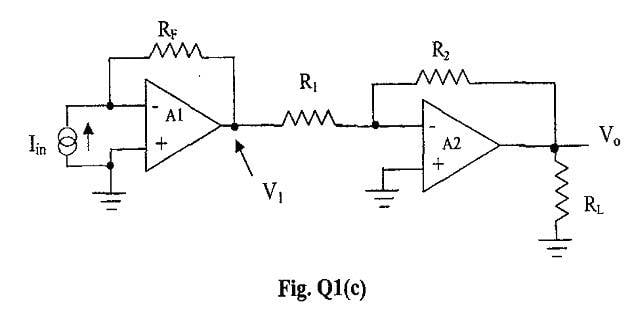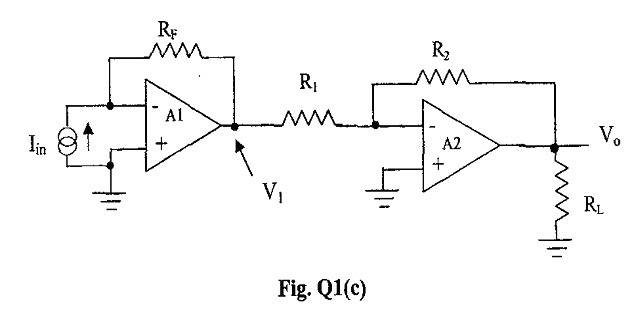# Ideal op amp (current to voltage converter + inverting)

naspek

## Homework Statement

Hey there.. need helps here..
The question is..
Derive an expression relating the output voltage, Vo to the input current, Iin.

## The Attempt at a Solution

i try to derive it.. but..
got problem with RL
V1 = -IinRf
Vo =- R2/R1(-IinRf) how to include Rl in my derivation?Mentor

## Homework Statement

Hey there.. need helps here..
The question is..
Derive an expression relating the output voltage, Vo to the input current, Iin.

## The Attempt at a Solution

i try to derive it.. but..
got problem with RL
V1 = -IinRf
Vo =- R2/R1(-IinRf) how to include Rl in my derivation?Good question. As long as the 2nd opamp's output is capable of driving RL, you don't need to include it in the gain equation. In real life, you would check the output current capability of the opamp, figure out the voltage swing based on your gain equation (for whatever values of Iin you expect), and make sure you weren't exceeding that output current rating.